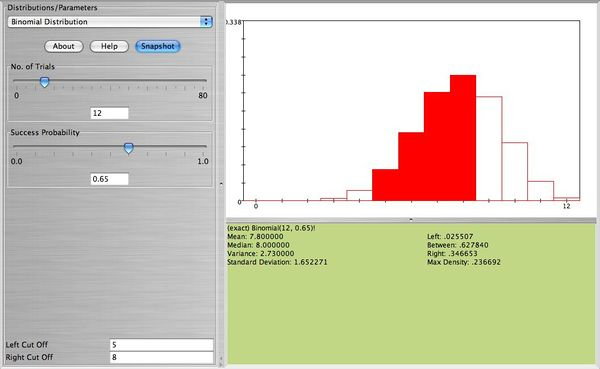# SOCR EduMaterials Activities More Examples

## SOCR Educational Materials - Activities - Solving Practical Problems using SOCR Distributions

This activity demonstrates solving practical problems using the SOCR Distribution Applets.

## Example 1

From a large shipment of peaches, 12 are selected for quality control. Suppose that in this particular shipment only $$65 \%$$ of the peaches are unbruised. If among the 12 peaches 9 or more are unbruised the shipment is classified A. If between 5 and 8 are unbruised the shipment is classified B. If fewer than 5 are unbruised the shipment is classified C. Compute the probability that the shipment will be classified A, B, C. Of course, computing the probabilities of two of these events yields the third probability, as $$1 = P(A \cup B \cup C) = P(A)+P(B)+P(C)$$.

We can use the Binomial distribution formula to compute

$$P(A) = P(X \ge 9) = \sum_{x=9}^{12} {12 \choose x} 0.65^x 0.35^{12-x}=\cdots$$

$$P(B) = P(5 \le X \le 8) = \sum_{x=5}^{8} {12 \choose x} 0.65^x 0.35^{12-x}=\cdots$$

$$P(C) = P(X < 5) = \sum_{x=0}^{4} {12 \choose x} 0.65^x 0.35^{12-x}=\cdots$$

Alternatively, we may solve the problem much easier using SOCR ...

Here is the distribution of the number of unbruised peaches among the 12 selected. After we enter $$n=12$$ and $$p=0.65$$ we get the distribution below:

Error creating thumbnail: File missing

Now, in the Left Cut Off and Right Cut Off boxes (bottom left corner of the applet) enter the numbers 5 and 8 respectively. What do you observe?The distribution is divided into three parts. The left part (less than 5), the right part (above 8), and the between part (between 5 and 8 included). All the SOCR distributions applets are designed in the same way. From the applet the probabilities are $$P(A)=0.346653, P(B)=0.627840, P(C)=0.025507.$$

## Example 2

Suppose a lot of size $$N$$ is accepted if it contains no more than $$c$$ defective components. A production manager selects at random a sample of $$n$$ components from this lot and determines the number of defective components. If he finds more than $$c$$ defective components then the lot is rejected, otherwise it is accepted. Answer the following questions:

a. Suppose the manager wants to choose between two lot sizes$N=500$ or $$N=1,000$$. Both lots will contain $$1 \%$$ defective components and he will sample in both cases $$n=5 \%$$ of the lot. Which sampling scheme will have a higher probability of rejecting the lot if $$c=0$$? Use SOCR and print the two snapshots.

b. Repeat (a) with $$c=1$$. Answer the question using SOCR.

c. Repeat (a) with $$c=1$$ and defective rate $$10 \%$$. Use SOCR.

## Example 3

The admissions office of a small, selective liberal-arts college will only offer admission to applicants who have a certain mix of accomplishments, including a high SAT score. Based on past records, the head of the admissions feels that the probability is 0.58 that an admitted applicant will come to the college. Based on financial considerations, the college would like a class of size 310 or more. Find the smallest $$n$$, number of people to admit, for which the probability of getting 310 or more to come to the college is at least 0.95. Use SOCR to find the answer and print the appropriate snapshots.

## Example 4

The diameters of apples from the Milo Farm have diameters that follow the normal distribution with mean 3 inches and standard deviation 0.3 inch. Apples can be size-sorted by being made to roll over a mesh screens. First, the apples are rolled over a screen with mesh size 2.5 inches. This separates out all the apples with diameters $$< 2.5$$ inches. Second, the remaining apples are rolled over a screen with mesh size 3.2 inches. Find the proportion of apples with diameters $$< 2.5$$ inches, between 2.5 and 3.2 inches, and greater than 3.2 inches. Use SOCR to find the answers and print the appropriate snapshots.